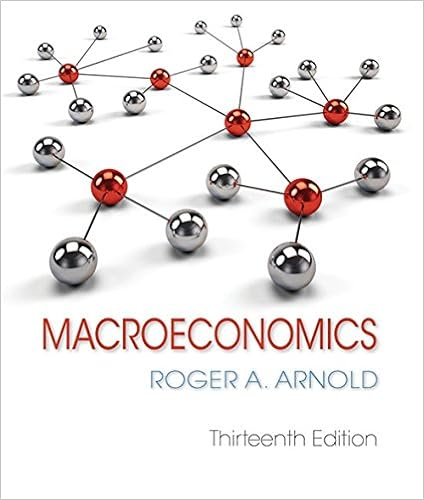# The aggregate demand curve tells us possible a

• 8
• 92% (12) 11 out of 12 people found this document helpful

This preview shows page 2 - 4 out of 8 pages.

Homework Set 8

The aggregate demand curve tells us possible A combinations of M and Y for a from ECO 3311 at Texas Tech University

6 .   The relationship between the quantity of output demanded and the aggregate price level is called :
A ) aggregate demand .
B ) aggregate supply .
C ) aggregate output .
D ) aggregate consumption .
7 .   If an aggregate demand curve is drawn with real GDP ( Y ) along the horizontal axis and the price level ( P ) along the vertical axis , using the quantity theory of money as a theory of aggregate demand , this curve slopes ______ to the right and gets ______ as it moves farther to the right .
8 .   The aggregate demand curve tells us possible :
9 .   The natural level of output is :
10 .   For a fixed money supply , the aggregate demand curve slopes downward because at a lower price level real money balances are ____ __ generating a ______ quantity of output demanded .
A ) higher ; greater
B ) higher ; smaller
C ) lower ; greater
D ) lower ; smaller
Answer:  a )   higher ; greater
11 .   When an aggregate demand curve is drawn with real GDP ( Y ) along the horizontal axis and the price level ( P ) along the vertical axis , if the money supply is decreased , then the aggregate demand curve will shift :
12 .   When the Federal Reserve reduces the money supply , at a given price level the amount of output demanded is ______ and the aggregate demand curve shifts _ _ ____.
13 .   When the Federal Reserve increases the money supply , at a given price level the amount of output demanded is ______ and the aggregate demand curve shifts _ _ ____.
14 .   The long run refers to a period :
B ) during which capital and labor are sometimes not fully employed .
C ) during which prices are flexible .
D ) during which output deviates from the full - employment level .
Answer:  C )   during which prices are flexible .
15 .   The short run refers to a period :
16 .   If a short - run equilibrium occurs at a level of output below the natural rate , then in the transition to the long run prices will ______ and output will _ _ ____.
##### We have textbook solutions for you!
The document you are viewing contains questions related to this textbook.The document you are viewing contains questions related to this textbook.
Chapter 13 / Exercise 5
Macroeconomics
Roger A. ArnoldExpert Verified
8.The aggregate demand curve tells us possible:A)combinations of Mand Yfor a given value of B)combinations of Mand Pfor a given value of C)combinations of Pand Yfor a given value of D)results if the Federal Reserve reduces the money supply.
P.
Y.
M.
9.The natural level of output is:
10.For a fixed money supply, the aggregate demand curve slopes downward because at a lower price level real money balances are ______ generating a ______ quantity of output demanded.
Page 2
##### We have textbook solutions for you!
The document you are viewing contains questions related to this textbook.The document you are viewing contains questions related to this textbook.
Chapter 13 / Exercise 5
Macroeconomics
Roger A. ArnoldExpert Verified
11.When an aggregate demand curve is drawn with real GDP (Y) along the horizontal axis and the price level (P) along the vertical axis, if the money supply is decreased, then the aggregate demand curve will shift:
12.When the Federal Reserve reduces the money supply, at a given price level the amount of output demanded is ______ and the aggregate demand curve shifts ______.A)greater; inwardB)greater; outwardC)lower; inwardD)lower; outward
13.When the Federal Reserve increases the money supply, at a given price level the amount of output demanded is ______ and the aggregate demand curve shifts ______.
14.The long run refers to a period:
•••